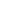# Use math drawings to compose a rectangle with square tiles

EngageNY 60 min(s)
In this lesson, students are introduced to composing a rectangle by using math drawings. Once students have arranged square tiles into a specified rectangular array without gaps or overlaps, they trace to construct the same rectangle by iterating the square unit. Next, students use the spatial reasoning developed up to this point in the module to draw the same rectangle without tracing, using their understanding of equal columns and equal rows.